# NCERT Solutions Chapter 8 - Quadrilaterals (II), Class 9, Maths Notes | Study Additional Documents & Tests for Class 9 - Class 9

## Class 9: NCERT Solutions Chapter 8 - Quadrilaterals (II), Class 9, Maths Notes | Study Additional Documents & Tests for Class 9 - Class 9

The document NCERT Solutions Chapter 8 - Quadrilaterals (II), Class 9, Maths Notes | Study Additional Documents & Tests for Class 9 - Class 9 is a part of the Class 9 Course Additional Documents & Tests for Class 9.
All you need of Class 9 at this link: Class 9

Exercise 8.2

1. ABCD is a quadrilateral in which P, Q, R and S are mid-points of the sides AB, BC, CD and DA (see Fig 8.29). AC is a diagonal. Show that :
(i) SR || AC and SR = 1/2 AC
(ii) PQ = SR
(iii) PQRS is a parallelogram.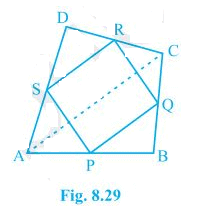(i) In ΔDAC,
R is the mid point of DC and S is the mid point of DA.
Thus by mid point theorem, SR || AC and SR = 1/2 AC

(ii) In ΔBAC,
P is the mid point of AB and Q is the mid point of BC.
Thus by mid point theorem, PQ || AC and PQ = 1/2 AC
also, SR = 1/2 AC
Thus, PQ = SR

(iii) SR || AC - from (i)
and, PQ || AC - from (ii)
⇒ SR || PQ - from (i) and (ii)
also, PQ = SR
Thus, PQRS is a parallelogram.

2. ABCD is a rhombus and P, Q, R and S are the mid-points of the sides AB, BC, CD and DA respectively. Show that the quadrilateral PQRS is a rectangle.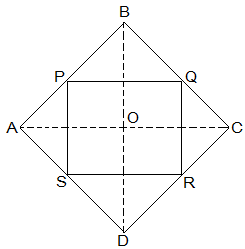Given,
ABCD is a rhombus and P, Q, R and S are the mid-points of the sides AB, BC, CD and DA respectively.
To Prove,
PQRS is a rectangle.
Construction,
AC and BD are joined.
Proof,
In ΔDRS and ΔBPQ,
DS = BQ (Halves of the opposite sides of the rhombus)
∠SDR = ∠QBP (Opposite angles of the rhombus)
DR = BP (Halves of the opposite sides of the rhombus)
Thus, ΔDRS ≅ ΔBPQ by SAS congruence condition.
RS = PQ by CPCT --- (i)
In ΔQCR and ΔSAP,
RC = PA (Halves of the opposite sides of the rhombus)
∠RCQ = ∠PAS (Opposite angles of the rhombus)
CQ = AS (Halves of the opposite sides of the rhombus)
Thus, ΔQCR ≅ ΔSAP by SAS congruence condition.
RQ = SP by CPCT --- (ii)
Now,
In ΔCDB,
R and Q are the mid points of CD and BC respectively.
⇒ QR || BD
also,
P and S are the mid points of AD and AB respectively.
⇒ PS || BD
⇒ QR || PS
Thus, PQRS is a parallelogram.
also, ∠PQR = 90°
Now,
In PQRS,
RS = PQ and RQ = SP from (i) and (ii)
∠Q = 90°
Thus, PQRS is a rectangle.

3. ABCD is a rectangle and P, Q, R and S are mid-points of the sides AB, BC, CD and DA respectively. Show that the quadrilateral PQRS is a rhombus.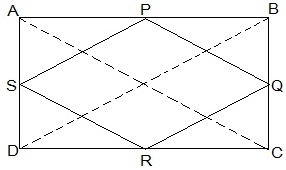Given,
ABCD is a rectangle and P, Q, R and S are mid-points of the sides AB, BC, CD and DA respectively.
Construction,
AC and BD are joined.
To Prove,
PQRS is a rhombus.
Proof,
In ΔABC
P and Q are the mid-points of AB and BC respectively
Thus, PQ || AC and PQ = 1/2 AC (Mid point theorem) --- (i)
SR || AC and SR = 1/2 AC (Mid point theorem) --- (ii)
So, PQ || SR and PQ = SR
As in quadrilateral PQRS one pair of opposite sides is equal and parallel to each other, so, it is a parallelogram.
PS || QR and PS = QR (Opposite sides of parallelogram) --- (iii)
Now,
In ΔBCD,
Q and R are mid points of side BC and CD respectively.
Thus, QR || BD and QR = 1/2 BD (Mid point theorem) --- (iv)
AC = BD (Diagonals of a rectangle are equal) --- (v)
From equations (i), (ii), (iii), (iv) and (v),
PQ = QR = SR = PS
So, PQRS is a rhombus.

4. ABCD is a trapezium in which AB || DC, BD is a diagonal and E is the mid-point of AD. A line is drawn through E parallel to AB intersecting BC at F (see Fig. 8.30). Show that F is the mid-point of BC.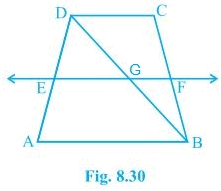Given,
ABCD is a trapezium in which AB || DC, BD is a diagonal and E is the mid-point of AD.
To prove,
F is the mid-point of BC.
Proof,
BD intersected EF at G.
E is the mid point of AD and also EG || AB.
Thus, G is the mid point of BD (Converse of mid point theorem)
Now,
In ΔBDC,
G is the mid point of BD and also GF || AB || DC.
Thus, F is the mid point of BC (Converse of mid point theorem)

5. In a parallelogram ABCD, E and F are the mid-points of sides AB and CD respectively (see Fig. 8.31). Show that the line segments AF and EC trisect the diagonal BD.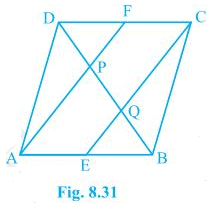Given,
ABCD is a parallelogram. E and F are the mid-points of sides AB and CD respectively.
To show,
AF and EC trisect the diagonal BD.
Proof,

ABCD is a parallelogram
Therefor, AB || CD

also, AE || FC
Now,
AB = CD (Opposite sides of parallelogram ABCD)
⇒ 1/2 AB = 1/2 CD
⇒ AE = FC (E and F are midpoints of side AB and CD)
AECF is a parallelogram (AE and CF are parallel and equal to each other)
AF || EC (Opposite sides of a parallelogram)
Now,
In ΔDQC,
F is mid point of side DC and FP || CQ (as AF || EC).
P is the mid-point of DQ (Converse of mid-point theorem)
⇒ DP = PQ --- (i)
Similarly,
In APB,
E is mid point of side AB and EQ || AP (as AF || EC).
Q is the mid-point of PB (Converse of mid-point theorem)
⇒ PQ = QB --- (ii)
From equations (i) and (i),
DP = PQ = BQ
Hence, the line segments AF and EC trisect the diagonal BD.

6. Show that the line segments joining the mid-points of the opposite sides of a quadrilateral bisect each other.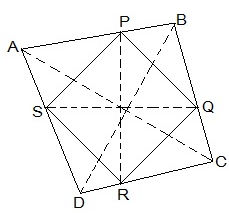Let ABCD be a quadrilateral and P, Q, R and S are the mid points of AB, BC, CD and DA respectively.
Now,
In ΔACD,
R and S are the mid points of CD and DA respectively.
Thus, SR || AC.
Similarly we can show that,
PQ || AC
PS || BD
QR || BD
Thus, PQRS is parallelogram.
PR and QS are the diagonals of the parallelogram PQRS. So, they will bisect each other.

7. ABC is a triangle right angled at C. A line through the mid-point M of hypotenuse AB and parallel to BC intersects AC at D. Show that
(i) D is the mid-point of AC
(ii) MD ⊥ AC
(iii) CM = MA = 1/2 AB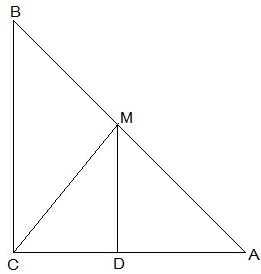(i) In ΔACB,
M is the mid point of AB and MD || BC
Thus, D is the mid point of AC (Converse of mid point theorem)

(ii) ∠ACB = ∠ADM (Corresponding angles)
also, ∠ACB = 90°
Thus, ∠ADM = 90° and MD ⊥ AC

(iii)  In ΔAMD and ΔCMD,
AD = CD (D is the midpoint of side AC)
DM = DM (common)
Thus, ΔAMD ≅ ΔCMD by SAS congruence condition.
AM = CM by CPCT
also, AM =  1/2 AB (M is mid point of AB)
Hence, CM = MA =  1/2 AB

The document NCERT Solutions Chapter 8 - Quadrilaterals (II), Class 9, Maths Notes | Study Additional Documents & Tests for Class 9 - Class 9 is a part of the Class 9 Course Additional Documents & Tests for Class 9.
All you need of Class 9 at this link: Class 9Use Code STAYHOME200 and get INR 200 additional OFF

## Additional Documents & Tests for Class 9

168 docs|11 tests

Track your progress, build streaks, highlight & save important lessons and more!

,

,

,

,

,

,

,

,

,

,

,

,

,

,

,

,

,

,

,

,

,

,

,

,

,

,

,

;